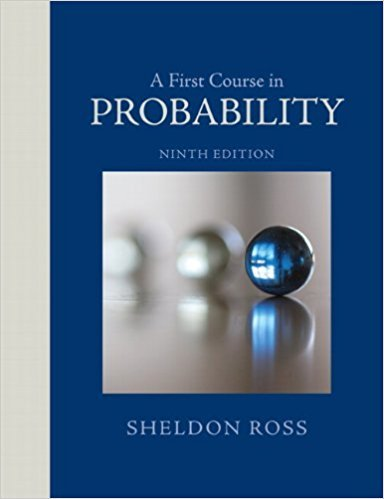×
Get Full Access to A First Course In Probability - 9 Edition - Chapter 1 - Problem 9ste
Get Full Access to A First Course In Probability - 9 Edition - Chapter 1 - Problem 9ste

×

# Consider three classes, each consisting of n students.ISBN: 9780321794772 63

## Solution for problem 9STE Chapter 1

A First Course in Probability | 9th Edition

• Textbook Solutions
• 2901 Step-by-step solutions solved by professors and subject experts
• Get 24/7 help from StudySoup virtual teaching assistantsA First Course in Probability | 9th Edition

4 5 1 421 Reviews
23
0
Problem 9STE

Problem 9STE

Consider three classes, each consisting of n students. From this group of 3n students, a group of 3 students is to be chosen.

(a) How many choices arc possible?

(b) How many choices arc there in which all 3 students are in the same class?

(c) How many choices are there in which 2 of the 3 students are in the same class and the other student is in a different class?

(d) How many choices are there in which all 3 students are in different classes?

(e) Using the results of parts (a) through (d), write a combinatorial identity.

Step-by-Step Solution:

Solution :

Step 1 of 5:

Given 3 classes, each consisting of n students.

Then from this group of 3n students, a group of 3 students is to be chosen.

a). From the given information we know that for the three classes, so each class consisting of n students.

Now we find the number of possible choices to a group of 3 students chosen from the group of 3n students inways.

Step 2 of 5

Step 3 of 5

##### ISBN: 9780321794772

Unlock Textbook Solution